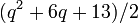# Number of conjugacy classes in projective symplectic group of fixed degree over a finite field is PORC function of field size

Jump to: navigation, search

## Statement

Suppose$n$ is an even natural number, i.e.,$n = 2m$ for some natural number$m$. Then, there exists a PORC function$f$ of degree$m = n/2$ such that, for any prime power$q$, the number of conjugacy classes in the projective symplectic group$PSp(n,q)$ (i.e., the projective symplectic group of degree$n$ over the finite field of size$q$) is$f(q)$.

A PORC function is a polynomial on residue classes -- it looks like different polynomial functions on different congruence classes modulo a particular number. In this case, we only need to consider congruence classes modulo$\operatorname{lcm}(2,m)$ to define the PORC function. In fact, for a field size of$q$, the polynomial depends only on the value$\operatorname{gcd}(\operatorname{lcm}(2,m),q - 1)$.

## Particular cases$n$ (degree of projective symplectic group)$m = n/2$ (degree of PORC function, also number used in Chevalley notation)$\operatorname{lcm}(2,m)$ Possibilities for$\operatorname{gcd}(\operatorname{lcm}(2,m),q - 1)$ Corresponding congruence classes mod$\operatorname{lcm}(2,m)$ for$q$ Corresponding polynomials in PORC function of$q$ giving number of conjugacy classes in$PSp(n,q)$ More information
2 1 2 1
2
0
1$q + 1$$(q + 5)/2$
See element structure of projective special linear group of degree two over a finite field (note that$PSp(2,q) = PSL(2,q)$).
4 2 2 1
2
0
1$q^2 + 2q + 3$$(q^2 + 6q + 13)/2$
element structure of projective symplectic group of degree four over a finite field